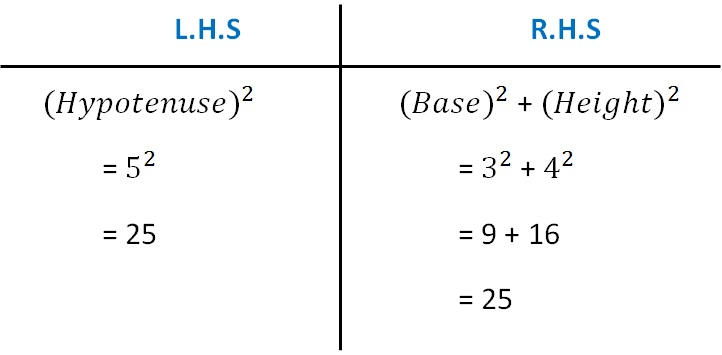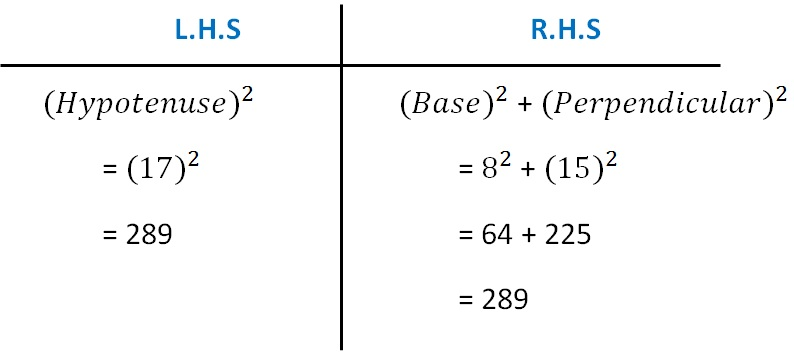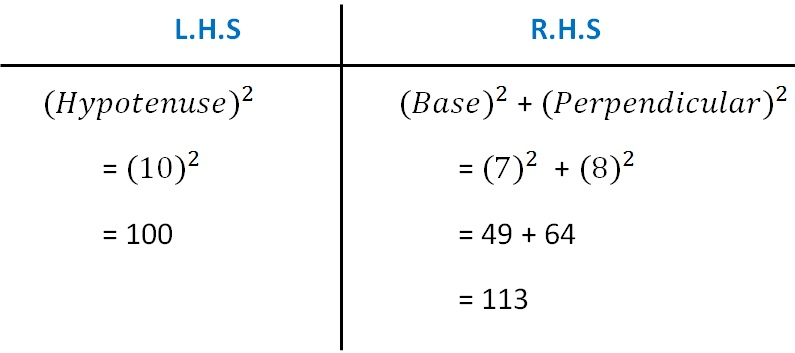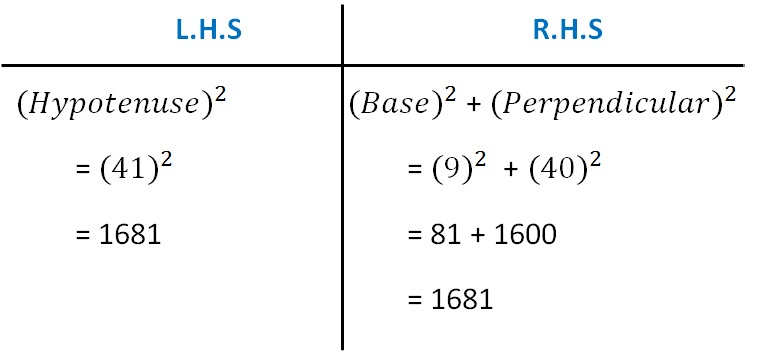Checking if triangle is right angled

Chapter 6 Class 7 Triangle and its Properties
Concept wise

Let’s take an example

Can 3, 4, 5 be the side of a right angled triangle?

We know that,

Hypotenuse is the longest side.

So, Hypotenuse = 5

Checking Pythagoras Theorem

(Hypotenuse) 2 = (Base) 2 + (Height) 2Since LHS = RHS,

Pythagoras Theorem is satisfied

Hence,

3, 4, 5 form sides of a right triangle,

with Hypotenuse = 5

Can 8, 15, 17 be the sides of a right angled triangle?

We know that,

Hypotenuse is the longest side.

So, Hypotenuse = 17

Checking Pythagoras Theorem

(Hypotenuse) 2 = (Base) 2 + (Perpendicular) 2Since L.H.S = R.H.S

Pythagoras theorem is satisfied

Hence,

8, 15, 17 form sides of a right triangle with

Hypotenuse = 17

Base = 8

Perpendicular = 15

Can 7, 8, 10 be the sides of a right angled triangle?

We know that,

Hypotenuse is the longest side.

So, Hypotenuse = 10

Checking Pythagoras Theorem

(Hypotenuse) 2 = (Base) 2 + (Perpendicular) 2Since L.H.S ≠ R.H.S

Hence,

7, 8, 10 do not form sides of a right triangle

Can 9, 40, 41 be the sides of a right angled triangle?

We know that,

Hypotenuse is the longest side.

So, Hypotenuse = 41

Checking Pythagoras Theorem

(Hypotenuse) 2 = (Base) 2 + (Perpendicular) 2Since L.H.S = R.H.S

Pythagoras theorem is satisfied

Hence,

9, 40, 41 form sides of a right triangle with

Hypotenuse = 41

Base = 9

Perpendicular = 40

Learn in your speed, with individual attention - Teachoo Maths 1-on-1 Class